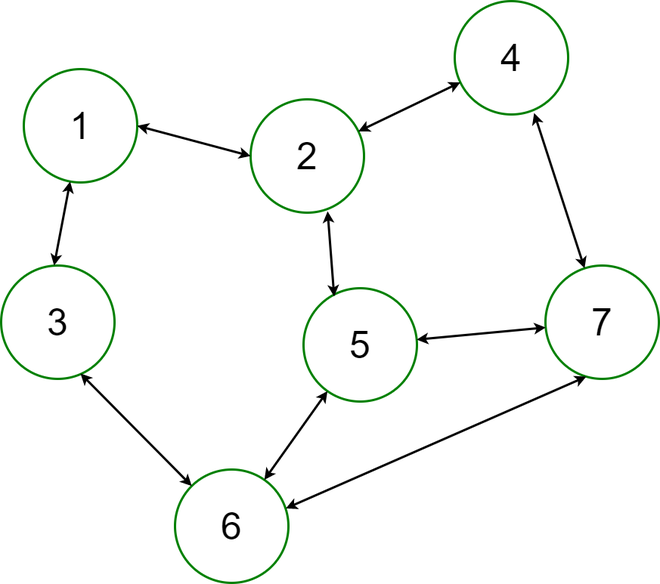Open In App

# How to Generate a Random Undirected Graph for a Given Number of Edges in Java?

An undirected graph is graph, i.e, a set of objects (called vertices or nodes) that are connected together, where all the edges are bidirectional. An undirected graph is sometimes called an undirected network. In contrast, a graph where the edges point in a direction is called a directed graph.Undirected Graph : Bidirectional

Examples:

```Input:
Enter the number of Edges :
5
Output:
The Generated Random Graph is :
1 -> { 5 2  }
2 -> { 1  }
3 -> { Isolated Vertex! }
4 -> { 6  }
5 -> { 1  }
6 -> { 4 10  }
7 -> { Isolated Vertex! }
8 -> { 10  }
9 -> { Isolated Vertex! }
10 -> { 8 6  }

Input:
Enter the number of Edges :
10
Output:
The Generated Random Graph is :
1 -> { 4 9  }
2 -> { 8  }
3 -> { 8  }
4 -> { 1 6  }
5 -> { 6  }
6 -> { 10 5 8 9 4  }
7 -> { 8  }
8 -> { 7 3 6 2  }
9 -> { 1 6  }
10 -> { 6  }```

Approach:

• Take the input of the number of edges for the random Directed Acyclic Graph.
• Build a connection between two random vertices iteratively.
• Assert “Isolated Vertex” if degree of a certain vertex is zero.

Implementation:

## Java

 `// Java program to Generate a Random Undirected Graph``// for a GivenNumber of Edges` `// importing generic packages``import` `java.util.*;``import` `java.util.Random;` `public` `class` `RandomUG {``    ``static` `int` `maxVertex = ``10``;` `    ``// Function to generate random graph``    ``public` `static` `void` `generateRandomGraphs(``int` `e)``    ``{``        ``int` `i = ``0``, j = ``0``, count = ``0``;` `        ``int``[][] edge = ``new` `int``[e][``2``];``        ``Random rand = ``new` `Random();` `        ``// Build a connection between two random vertex``        ``while` `(i < e) {``            ``edge[i][``0``] = rand.nextInt(maxVertex) + ``1``;``            ``edge[i][``1``] = rand.nextInt(maxVertex) + ``1``;` `            ``// using rand to pick a random integer in range``            ``// of (1 - maxVertex)``            ``i++;``        ``}` `        ``System.out.println(``            ``"The Generated Random Graph is :"``);` `        ``// Print the Graph``        ``for` `(i = ``0``; i < maxVertex; i++) {``            ``count = ``0``;` `            ``System.out.print((i + ``1``) + ``" -> { "``);` `            ``for` `(j = ``0``; j < e; j++) {``                ``if` `(edge[j][``0``] == i + ``1``) {``                    ``System.out.print(edge[j][``1``] + ``" "``);``                    ``count++;``                ``}` `                ``else` `if` `(edge[j][``1``] == i + ``1``) {``                    ``System.out.print(edge[j][``0``] + ``" "``);``                    ``count++;``                ``}` `                ``// print “Isolated vertex” for the vertex``                ``// having zero degree.``                ``else` `if` `(j == e - ``1` `&& count == ``0``)``                    ``System.out.print(``"Isolated Vertex!"``);``            ``}``            ``System.out.print(``" }\n"``);``        ``}``    ``}` `    ``public` `static` `void` `main(String args[]) ``throws` `Exception``    ``{``        ``int` `e = ``6``;``        ``System.out.println(``"Enter the number of Edges : "` `+e);` `        ``// Function to generate a Random unDirected Graph``        ``generateRandomGraphs(e);``    ``}``}`

Output

```Enter the number of Edges : 6
The Generated Random Graph is :
1 -> { 8  }
2 -> { 2  }
3 -> { Isolated Vertex! }
4 -> { 5  }
5 -> { 4 6  }
6 -> { 5  }
7 -> { 9  }
8 -> { 1 10  }
9 -> { 7  }
10 -> { 8  }```